FreeBuf+小程序2015-09-22 09:00:590x01 检测思路

```一、Form表单里如果存在img标签，则判断src属性值，查看后缀是是否为jpg、png、jpeg、gif，如果不为这些后缀，说明是“验证码”返回false，因为存在“验证码”的form表单，ajax发送会出错，因为我们不知道获取“验证码”的值。

0x02 测试环境

```一、需要一个form表单里存在图片，而且可以触发XSS。

```<form method="post">
<input type="text" name="xss">
<img src="https://image.3001.net/images/new/logo.png" alt="">
<input type="submit">
</form><br><hr>```

```<form method="post" action="http://baidu.com/">
<input type="text" name="xss">
<img src="http://loudong.360.cn/images/logo.png" alt="">
<input type="submit">
</form><br><hr>```

```<form action="#" method="post">
<input type="text" name="xss">
<input type="submit">
</form><br><hr>```

```<form  method="get">
<input type="text" name="xss">
<input type="text" name="caonima">
<input type="submit">
</form><br><hr>```

```<form  method="get" action="http://360.cn/">
<input type="text" name="asd">
<input type="text" name="wocaonimabi">
<input type="submit">
</form>```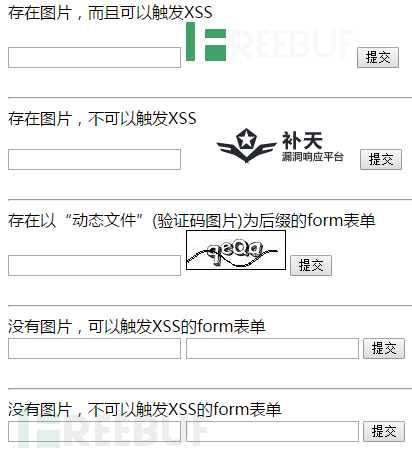```<?php
if(isset(\$_POST['xss'])){
echo \$_POST['xss'];
}
if(isset(\$_GET['a'])){
echo \$_GET['a'];
}
if(isset(\$_GET['caonima'])){
echo \$_GET['caonima'];
}
?>```

0x03 函数变量

```var tureForm;     //存放符合条件的form表单数据
var tureInput;        //存放符合条件的input标签数据
var formImg;      //form表单里的img标签里的src属性值后缀
var actionUrl;        //form表单的action属性的值
var methodType;   //form表单的method属性的值
var sendDataUrl;  //发送时的URL
var i;             //第一层for循环的初始变量
var j;             //第二层for循环的初始变量```

0x04 form表单筛选

```var numbers = [1,2,3,4,5];
filterNubmbers = numbers.filter(function(item,index){
return (item > 3)
})```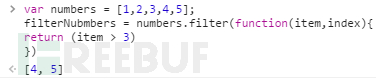item是数组里的项，index是项在数组里的位置。

fliter函数是提供给Array的，也就是Array.fliter(function(item,index){...})，但是也可以使用Object。也就是Object.filter(function(item,index){...})

`tureForm = \$("form").filter(function(item,index){});`

Jquery返回的是“Object对象”这点切记，也就是说什么的filter其实是对象。那item和index就已经互换了。下面的index就是数组里的项，也就是页面中每一个form表单数据。我们打印看下：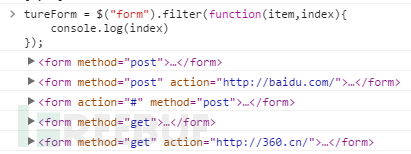`formImg = \$(index).find("img").attr("src");`

forImg现在就等于img标签里src的值了。下面就是做判断是否存src这个属性：

```if(!!formImg){    /*当存在src属性时，运行代码*/ }else{
/*当不存在src属性时，运行的代码*/
}```

!!的作用将后面的forImg强制转换为布尔类型的数据。方便if判断。

`http://www.freebuf.com/logo.png?rand=012350154453`

```if(formImg.indexOf("?") != "-1"){
formImg = formImg.slice(0,formImg.indexOf("?"));
}```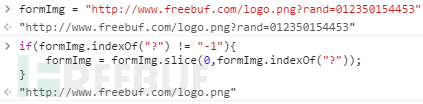`formImg = formImg.substr(formImg.lastIndexOf("."),formImg.length);`

formImg.lastIndexOf(".")是substr函数的第一个参数，计算formImg字符串从后开始第一个"."的下标位置，为什么不用indexOf呢，因为去掉参数后，后缀也就只有几个字符，而前面则是非常多的字符串。这样一来，速度快一些。获取从最后开始出现的"."字符。而且url地址都会有.。比如freebuf.com或者127.0.0.1这样获取的字符串就不准确了。

formImg.length则是formImg变量的长度，也就是去掉参数的图片地址有多少个字符串。

formImg = formImg.substr(formImg.lastIndexOf("."),formImg.length);这一段代码就完成了获取后缀的功能，我们开输出看下：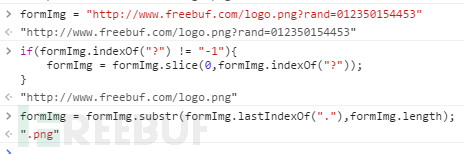```if(formImg == ".png" || formImg == ".jpg" || formImg == ".jpeg" || formImg == ".gif"){
/*当符合上面的条件时，运行代码*/
}```

||管道符的作用是，当前为false时，再判断后面的。说详细点就是：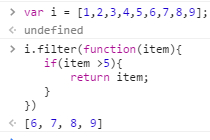`return \$(index).find(":submit").length > 0 && (\$(index).find(":text").length > 0 || \$(index).find(":password").length || \$(index).find(":radio").length > 0 || \$(index).find(":checkbox").length > 0);`

OK，这个时候存在图片的判断已经写完了，那当前的form表单不存在图片怎么办呢？这个时候我们就不需要那么多的if判断图片格式了。我就直接在else里写上：

`return \$(index).find(":submit").length > 0 && (\$(index).find(":text").length > 0 || \$(index).find(":password").length || \$(index).find(":radio").length > 0 || \$(index).find(":checkbox").length > 0);`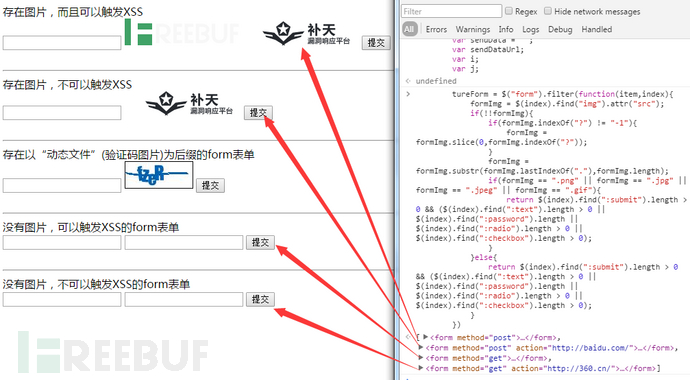```if(tureForm.length <= 0){
return false;
}```

```tureForm = \$(tureForm).filter(function(item,index){
return (!!\$(index).find(":input").attr("name"));
})
if(tureForm.length <= 0){
return false;
}```

filter这段代码完成的功能就是，把当前的form表单重新组合，把具有name属性的input标签组成新的form表单，把不存在name属性的标签给剔除掉。

```tureForm = \$("form").filter(function(item,index){
formImg = \$(index).find("img").attr("src");
if(!!formImg){
if(formImg.indexOf("?") != "-1"){
formImg = formImg.slice(0,formImg.indexOf("?"));
}
formImg = formImg.substr(formImg.lastIndexOf("."),formImg.length);
if(formImg == ".png" || formImg == ".jpg" || formImg == ".jpeg" || formImg == ".gif"){
return \$(index).find(":submit").length > 0 && (\$(index).find(":text").length > 0 || \$(index).find(":password").length || \$(index).find(":radio").length > 0 || \$(index).find(":checkbox").length > 0);
}
}else{
return \$(index).find(":submit").length > 0 && (\$(index).find(":text").length > 0 || \$(index).find(":password").length || \$(index).find(":radio").length > 0 || \$(index).find(":checkbox").length > 0);
}
})
if(tureForm.length <= 0){
return false;
}
tureForm = \$(tureForm).filter(function(item,index){
return (!!\$(index).find(":input").attr("name"));
})
if(tureForm.length <= 0){
return false;
}```

0x05 发送数据

```for(i = 0;i < tureForm.length;i++){
/*tureForm[i]代表当前的form表单*/
}```

```actionUrl = \$(tureForm[i]).attr("action");
methodType = \$(tureForm[i]).attr("method");```

actionUrl代表了当前form表单的action字符串，methodType同理。

```if(actionUrl == undefined || actionUrl == "#" || actionUrl == ""){
actionUrl = href;
}
if(methodType == undefined || methodType == ""){
methodType = "get";
}```

`tureInput = \$(tureForm[i]).find("input:not(:submit)").length;`

```for(j = 0;j < tureInput;j++){
sendData += \$(tureForm[i]).find("input:not(:submit)")[j].getAttribute("name") + "=" + onlyString + j + "&";
}```

sendData是拼凑后的字符，至于为什么这里不用attr，而是用getAttribute这种原生态JavaScript，因为前面的[j]就已经把前面的jQuery对象转成原生态的JavaScript了，想要使用attr也可以，这样写：

`\$(\$(tureForm[i]).find("input:not(:submit)")[j]).getAttribute("name")`

onlyString + j就是当前这个input的唯一标识符了。

`sendDataUrl = sendData.substring(0,sendData.length-1);`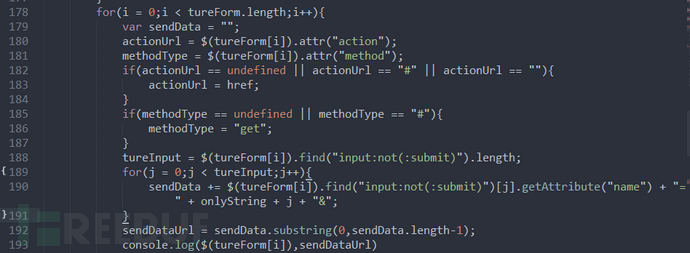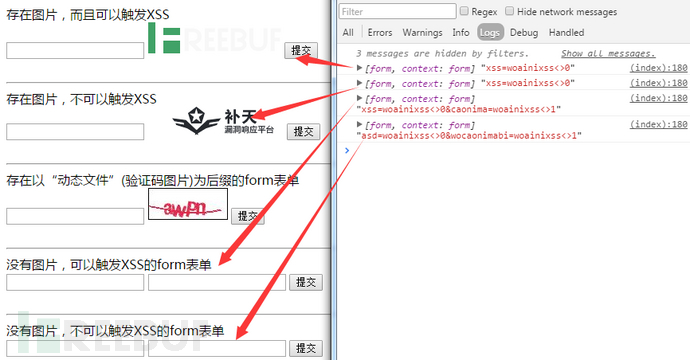```\$.ajax({
url: actionUrl,
type: methodType,
dataType: 'text',
data: sendDataUrl,
async:false,
})```

Url是action发送地址，type是method发送模式，data就是拼凑的数据。

async:false是以异步发送，防止for+ajax的bug。

```.done(function(data){
/*data为发送成功后，服务端的反馈的数据*/
})```

```for(j = 0;j < tureInput;j++){
if(data.indexOf(onlyString + j) != "-1"){
xss += j + 1 + "|";
}
}```

If判断当前form发送的数据里存不存在XSS。这里的onlyString + j和上面拼接的数据是一样的，不然无法判断，当data.indexOf(onlyString + j) != "-1"的结果为true时，说明存在XSS，这个时候我们在把存在XSS的数据，放到xss变量里。

```if(xss == ""){
return false;
}else{
/*存在XSS时，要运行的代码*/
}```

`xss = xss.substring(0,xss.length-1);`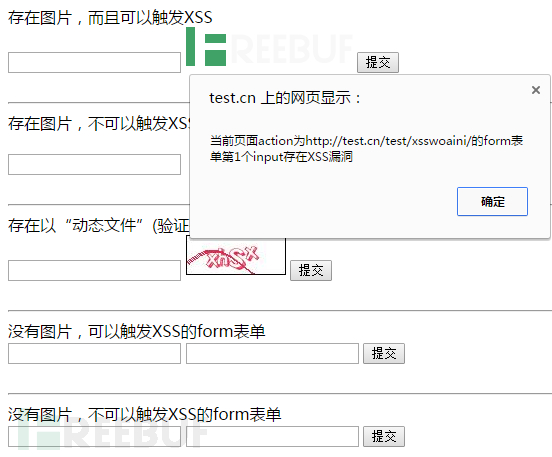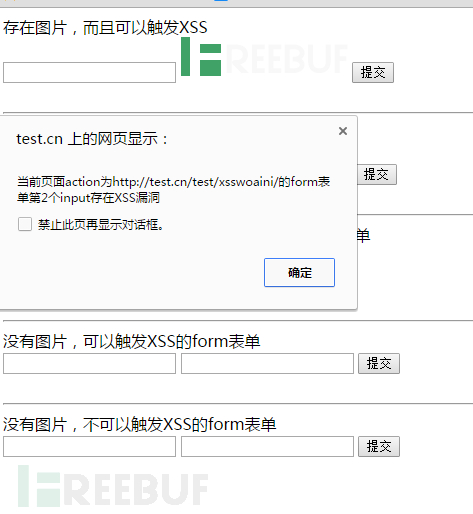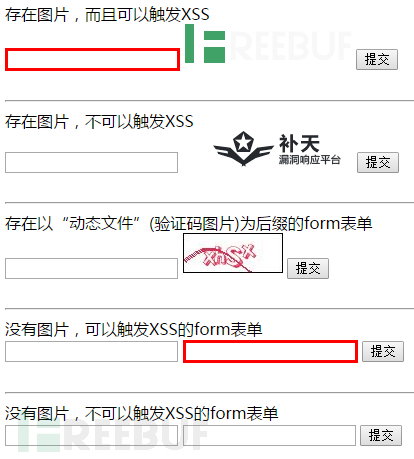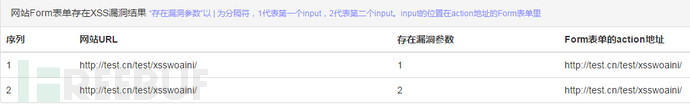```for(i = 0;i < tureForm.length;i++){
var sendData = "";
actionUrl = \$(tureForm[i]).attr("action");
methodType = \$(tureForm[i]).attr("method");
if(actionUrl == undefined || actionUrl == "#" || actionUrl == ""){
actionUrl = href;
}
if(methodType == undefined || methodType == "#"){
methodType = "get";
}
tureInput = \$(tureForm[i]).find("input:not(:submit)").length;
for(j = 0;j < tureInput;j++){
sendData += \$(tureForm[i]).find("input:not(:submit)")[j].getAttribute("name") + "=" + onlyString + j + "&";
}
sendDataUrl = sendData.substring(0,sendData.length-1);
\$.ajax({
url: actionUrl,
type: methodType,
dataType: 'text',
data: sendDataUrl,
async:false,
})
.done(function(data) {
var xss = "";
for(j = 0;j < tureInput;j++){
if(data.indexOf(onlyString + j) != "-1"){
xss += j + 1 + "|";
}
}
if(xss == ""){
return false;
}else{
xss = xss.substring(0,xss.length-1);
// \$(tureForm[i]).find("input").eq(xss - 1).css("border"," 3px solid red");
// alert("当前页面action为" + actionUrl + "的form表单第" + xss + "个input存在XSS漏洞");
\$("body").append("<img src='http://xss.cn/formXSS.html?host=\$" + href + "&\$xss=\$" + xss + "&\$url=\$" +actionUrl + "&\$rand=\$" + Date.parse(new Date()) + "' style='display:none;'>");
}
})
}```

0x06 重写反馈

```<style>
.hide{
display: none;
}
.show{
display: inline-table !important;
}
small{
color: #898FFF;
}
</style>```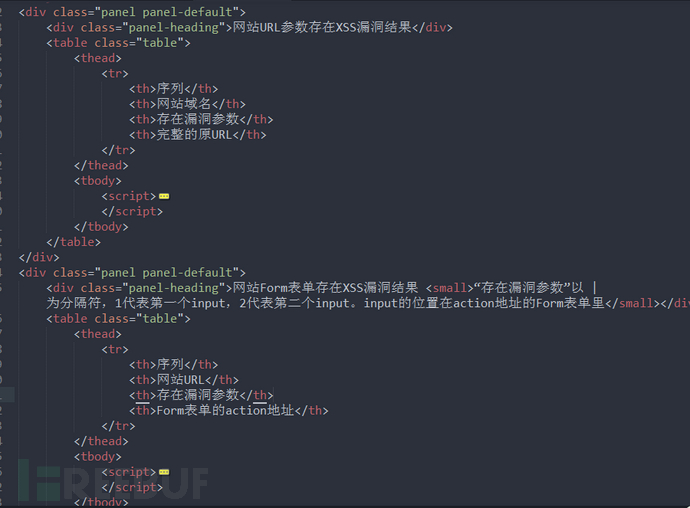Script里就是处理数据的，和之前的是没什么太大变化。

```<script>
if(\$(this).next("table").attr("class") == "table hide"){
\$(this).next("table").removeClass('hide');
}else{
\$(this).next("table").removeClass('show');
}
});
</script>```

```<!DOCTYPE html>
<html>
<meta charset="utf-8">
<meta http-equiv="X-UA-Compatible" content="IE=edge">
<title>XSS反馈</title>
<script src="http://apps.bdimg.com/libs/jquery/2.1.4/jquery.js"></script>
<style>
.hide{
display: none;
}
.show{
display: inline-table !important;
}
small{
color: #898FFF;
}
</style>
<body>
<div class="panel panel-default">
<div>网站URL参数存在XSS漏洞结果</div>
<table>
<tr>
<th>序列</th>
<th>网站域名</th>
<th>存在漏洞参数</th>
<th>完整的原URL</th>
</tr>
<tbody>
<script>
\$.ajax({
url: '/logs/getXSS.log',
type: 'get',
dataType: 'text',
})
.done(function(data) {
var host = "";
var xss = "";
var url = "";
var htmlText = "";
data = data.split("\n");
if(data[data.length-1] == ""){
data.pop();
}
for(var i = 0;i < data.length;i++){
data[i] = data[i].substring(15);
data[i] = data[i].substring(0,data[i].length-11);
}
for(i = 0;i<data.length;i++){
data[i] = data[i].split("&\$");
host += data[i].split("=\$") + " ";
xss += data[i].split("=\$") + " ";
url += data[i].split("=\$") + " ";
}
host = host.split(" ");
host.pop();
xss = xss.split(" ");
xss.pop();
url = url.split(" ");
url.pop();
for(i = 0;i < data.length;i++){
htmlText += "<tr><td>"+ (i+1) +"</td><td>" + host[i] + "</td><td>" + xss[i] + "</td><td>" + url[i] + "</td></tr>";
}
\$(\$("tbody")).append(htmlText);
})
</script>
</tbody>
</table>
</div>
<div class="panel panel-default">
<div>网站Form表单存在XSS漏洞结果 <small>“存在漏洞参数”以 | 为分隔符，1代表第一个input，2代表第二个input。input的位置在action地址的Form表单里</small></div>
<table>
<tr>
<th>序列</th>
<th>网站URL</th>
<th>存在漏洞参数</th>
<th>Form表单的action地址</th>
</tr>
<tbody>
<script>
\$.ajax({
url: '/logs/formXSS.log',
type: 'get',
dataType: 'text',
})
.done(function(data) {
var host = "";
var xss = "";
var url = "";
var htmlText = "";
data = data.split("\n");
if(data[data.length-1] == ""){
data.pop();
}
for(var i = 0;i < data.length;i++){
data[i] = data[i].substring(15);
data[i] = data[i].substring(0,data[i].length-11);
}
for(i = 0;i<data.length;i++){
data[i] = data[i].split("&\$");
host += data[i].split("=\$") + " ";
xss += data[i].split("=\$") + " ";
url += data[i].split("=\$") + " ";
}
host = host.split(" ");
host.pop();
xss = xss.split(" ");
xss.pop();
url = url.split(" ");
url.pop();
for(i = 0;i < data.length;i++){
htmlText += "<tr><td>"+ (i+1) +"</td><td>" + host[i] + "</td><td>" + xss[i] + "</td><td>" + url[i] + "</td></tr>";
}
\$(\$("tbody")).append(htmlText);
})
</script>
</tbody>
</table>
</div>
<script>
if(\$(this).next("table").attr("class") == "table hide"){
\$(this).next("table").removeClass('hide');
}else{
\$(this).next("table").removeClass('show');
}
});
</script>
</body>
</html>```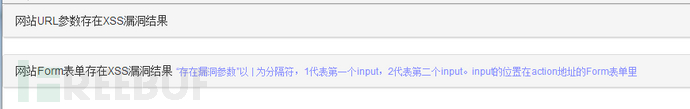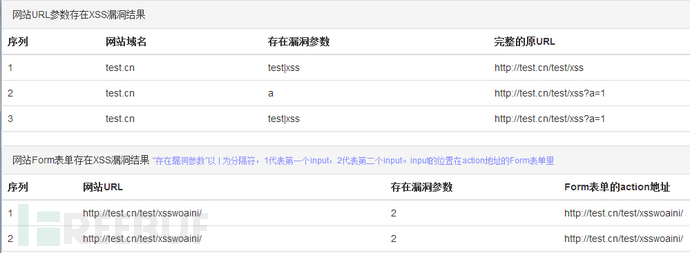Chrome：上传插件还要交费，所以我就没上传到chroem应用平台

*本文来自FreeBuf特约作者Black-Hole投稿，属FreeBuf黑客与极客（Freebuf.COM）独家发布，未经允许禁止转载。

# xss

+ 收入我的专栏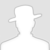• 0 文章数
• 0 评论数
• 0 关注者